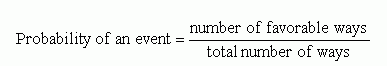Email us to get an instant 20% discount on highly effective K-12 Math & English kwizNET Programs!

#### Online Quiz (WorksheetABCD)

Questions Per Quiz = 2 4 6 8 10

### Grade 4 - Mathematics10.1 Probability

 Probability Is the chance of an event happening. If a particular outcome can never occur, its probability is 0. If an outcome is certain to occur, its probability is 1. In general, if p is the probability that a specific outcome will occur, values of p fall in the range 0 <= p <= 1. Probability may be expressed as either a decimal, a fraction, or a ratio. Probability examples in everyday life include physical sciences, commerce, weather forecasting, board games, sports statistics, etc. Examples: What are the chances that the sun will shine this month? (likely) What are the chances that you, a 11 year old boy, will be driving a car today? (unlikely) What are the chances of tossing heads or tails on a coin? (equally likely)Coin: 2 sides The probability of rolling heads is 1/2 The probability of rolling tails is 1/2 Cube: 6 sides Take a number cube and label the sides 1 - 6. The probability of rolling a 3 is one outcome out of a total of six possible outcomes, that is 1/6. Similarly, the probability of rolling a 5 is also 1/6. The probability of rolling an odd number (1, 3, 5) = three outcomes out of a total of six possible outcomes = 3/6 = 1/2 Probability of rolling an even number is also same as rolling an odd numbers (3/6 or 1/2). Example: A bag contains 4 red balls and 3 green balls. What is the probability of picking a green ball? Since there are three green and 1 is picked out at a time, the probability is 3/7. Answer: 3/7 Directions: Solve the following questions. Also write at least 10 examples of your own.
 Q 1: A spinner with 8 sections of equal size with 1 through 8 numbers on them. What is the probability of Melissa getting a 3 or a 5?3/81/82/8 Q 2: There are 4 balls of the same size in a container. 2 are red, 1 is green and 1 is blue. Dan reaches in and draws out 1 ball. What is the probability that it is blue ball?1 out of 42 out of 41 out of 3 Q 3: When you flip a coin what is the probability of getting heads?1/11/31/2 Q 4: There are 4 balls of the same size in a container. 2 are red, 1 is green and 1 is blue. Dan reaches in and draws out 1 ball. What is the probability that it is red ball?2 out of 41 out of 41 out of 3 Question 5: This question is available to subscribers only! Question 6: This question is available to subscribers only!